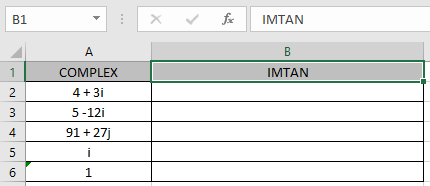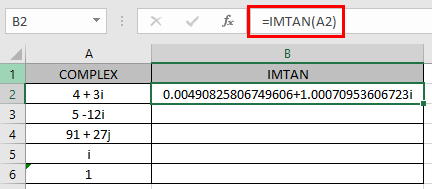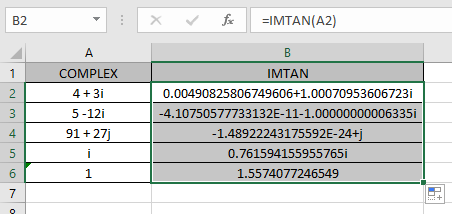# How to use the IMTAN Function in Excel

COMPLEX number (inumber) in excel derived for mathematical number having real and imaginary coefficients. In mathematics we call it the coefficient of i or j (iota).
i = √-1

Square root of negative number is not possible, so for calculation purpose, √-1 is named as imaginary and call it iota (i or j). For calculation of some term like shown below.

= 2 +√-25

= 2 +√-1*25

= 2 +√-1*25

= 2 +√-1* 5

= 2 + 5i

This here equation is a Complex number (inumber) having 2 different parts called real part imaginary part

The coefficient of iota (i) which is 5 is called as imaginary part and the other part 2 is called the real part of the complex number.

Complex number (inumber) is written in the X iY format.

Complex tangent of a complex number (X + iY) is given by

Tan (X + iY) Sin (X + iY) / Cos (X + iY)

So to calculate the above equation we will use the below two equations.
Sin (X + iY) Sin (X) * Cosh (Y) + i Cos (X) * Sinh (Y)

Cos (X + iY) Cos (X) * Cosh (Y) - i Sin (X) * Sinh (Y)

Here:

1. Tan is the tangent function
2. Cos is the Cosine function
3. Sin is the Sine function
4. Cosh is the hyperbolic Sine function
5. Sinh is the hyperbolic Sine function

Here X & Y are the coefficients of the real & imaginary part of the complex number (inumber).

The IMTAN function returns the complex tangent of the complex number (inumber) having both real & imaginary part.

Syntax:

=IMTAN (inumber)

inumber : complex number having both real & imaginary

Let’s understand this function using it in an example.Here we have values where we need to get the complex Sine of the input complex number (inumber)

Use the formula:

=IMTAN (A2)

A2 : complex number (inumber) provided as cell reference.As you can see the complex number having real_num = 4 & imaginary part = 3. The formula returns the complex Sine of the complex number using the following formulation.
Sin (4 + 3i) Sin (4) * Cosh (3) + i Cos (4) * Sinh (3)
Cos (4 + 3i) = Cos (4) * Cosh (3) - i Sin (4) * Sinh (3)

Tan (4 + 3i) = Sin (4 + 3i) / Cos (4 + 3i)

Now copy the formula to the other remaining cells using Ctrl + D shortcut key.As you can see the IMTAN function formula giving results just fine.

The table show here explains more about the input complex number & how excel reads it.

 inumber Real part (X) Imaginary part (Y) i = 0 + 1i 0 1 1 = 1 + 0i 1 0

Note :

1. The formula returns the #NUM! error if the complex number doesn’t have lower case i or j (iota).
2. The function returns the #VALUE! Error if the input argument to the function is a logical value.
3. The input inumber or complex number must be in the X + Yi text format.

Hope you understood how to use IMTAN function and referring cell in Excel. Explore more articles on Excel mathematical functions here. Please feel free to state your query or feedback for the above article.

Related Articles
How to use the TYPE function
How to use the CELL function

Terms and Conditions of use

The applications/code on this site are distributed as is and without warranties or liability. In no event shall the owner of the copyrights, or the authors of the applications/code be liable for any loss of profit, any problems or any damage resulting from the use or evaluation of the applications/code.